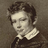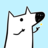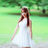首页   注册   登录
V2EX = way to explore
V2EX 是一个关于分享和探索的地方

Udacity

Godel, Escher, Bach: An Eternal Golden BraidV2EX  ›  分享发现

# 汉语中的词频及笔画数分布规律探析

metaquant · 2018-09-15 12:59:11 +08:00 · 1325 次点击

## 一、引言## 二、汉语词频分布规律

import numpy as np
import statsmodels.api as sm
import matplotlib.pyplot as plt
import warnings
warnings.filterwarnings("ignore")
import seaborn as sns
sns.set(style="white",color_codes=True)
import pandas as pd
%matplotlib inline
plt.rcParams['figure.figsize'] = (15,9.27)


df = pd.read_excel('word_freq.xlsx')

rank word freq freq_percent cum_freq_percent
0 1 744863 7.7946 7.7946
1 2 130191 1.3624 9.1570
2 3 118823 1.2434 10.4004
3 4 118527 1.2403 11.6407
4 5 83958 0.8786 12.5193
5 6 81119 0.8489 13.3682
6 7 65146 0.6817 14.0499
7 8 53556 0.5604 14.6103
8 9 52912 0.5537 15.1640
9 10 52728 0.5518 15.7158

df['log_rank'] = np.log10(df['rank'])
df['log_freq'] = np.log10(df['freq'])
df['word_count'] = df['word'].apply(len)

rank word freq freq_percent cum_freq_percent log_rank log_freq word_count
0 1 744863 7.7946 7.7946 0.000000 5.872076 1
1 2 130191 1.3624 9.1570 0.301030 5.114581 1
2 3 118823 1.2434 10.4004 0.477121 5.074901 1
3 4 118527 1.2403 11.6407 0.602060 5.073817 1
4 5 83958 0.8786 12.5193 0.698970 4.924062 1
5 6 81119 0.8489 13.3682 0.778151 4.909123 1
6 7 65146 0.6817 14.0499 0.845098 4.813888 1
7 8 53556 0.5604 14.6103 0.903090 4.728808 1
8 9 52912 0.5537 15.1640 0.954243 4.723554 1
9 10 52728 0.5518 15.7158 1.000000 4.722041 1

word_count列，我们可以看到，词频表中收录的最长的词包含十个单字，平均而言包含两个单字。通过简单的统计，我们可以看到词语中汉字个数的分布规律如下表：其中两字词出现的次数最多，为 10476 次，占比达到 71.61%；其次为单字词，为 2391 次，占比达到 16.34%。

# Count the frequency and frequency of occurrences of words of various lengths

word_length = pd.DataFrame(df.word_count.value_counts()).sort_index()
word_length.columns = ['freq']
word_length['freq_percent'] = 100*word_length['freq']/sum(word_length['freq'])

freq freq_percent
1 2391 16.344248
2 10476 71.611183
3 1181 8.073006
4 506 3.458883
5 43 0.293937
6 22 0.150386
7 8 0.054686
8 1 0.006836
10 1 0.006836

sns.regplot(df.log_rank,df.log_freq)
plt.xlim(0,4.3)
plt.ylim(1,7)
plt.title('The relationship between the logarithm of the frequency ranking and the logarithm of the frequency')def reg(y,*args):
import statsmodels.api as sm
x = np.vstack((args)).T
res = sm.OLS(y,mat_x).fit()
print(res.summary())

reg(df.log_rank,df.log_freq)

                            OLS Regression Results
==============================================================================
Dep. Variable:               log_rank   R-squared:                       0.990
Method:                 Least Squares   F-statistic:                 1.502e+06
Date:                Sat, 15 Sep 2018   Prob (F-statistic):               0.00
Time:                        10:27:07   Log-Likelihood:                 25420.
No. Observations:               14629   AIC:                        -5.084e+04
Df Residuals:                   14627   BIC:                        -5.082e+04
Df Model:                           1
Covariance Type:            nonrobust
==============================================================================
coef    std err          t      P>|t|      [0.025      0.975]
------------------------------------------------------------------------------
const          5.7144      0.002   3450.432      0.000       5.711       5.718
x1            -0.8846      0.001  -1225.535      0.000      -0.886      -0.883
==============================================================================
Omnibus:                    15756.722   Durbin-Watson:                   0.007
Prob(Omnibus):                  0.000   Jarque-Bera (JB):          2257816.630
Skew:                          -5.242   Prob(JB):                         0.00
Kurtosis:                      62.952   Cond. No.                         12.8
==============================================================================

Warnings:
 Standard Errors assume that the covariance matrix of the errors is correctly specified.


sns.residplot(df.log_rank,df.log_freq)
plt.ylabel('resid')
plt.title('The relationship between the residual and the logarithm of the frequency ranking')log_rank_first = df.log_rank[df.freq>=296]
log_freq_first = df.log_freq[df.freq>=296]
log_rank_second = df.log_rank[(126<=df.freq) & (df.freq<296)]
log_freq_second = df.log_freq[(126<=df.freq) & (df.freq<296)]
log_rank_third = df.log_rank[(51<df.freq) & (df.freq<126)]
log_freq_third = df.log_freq[(51<df.freq) & (df.freq<126)]
log_rank_fourth = df.log_rank[df.freq<=51]
log_freq_fourth = df.log_freq[df.freq<=51]

plt.subplot(221)
sns.regplot(log_rank_first,log_freq_first)
plt.title('$frequency \geq 296,R^2=0.992$')
plt.xlabel('')
plt.subplot(222)
sns.regplot(log_rank_second,log_freq_second)
plt.title('$126 \leq frequency \leq 296,R^2=0.999$')
plt.xlabel('')
plt.ylabel('')
plt.subplot(223)
sns.regplot(log_rank_third,log_freq_third)
plt.title('$51<frequency<126,R^2=1.000$')
plt.subplot(224)
sns.regplot(log_rank_fourth,log_freq_fourth)
plt.title('$frequency \leq 51,R^2=0.000$')
plt.ylabel('')plt.subplot(221)
sns.residplot(log_rank_first,log_freq_first)
plt.title('$frequency \geq 296,R^2=0.992$')
plt.xlabel('')
plt.ylim(-1,0.2)
plt.subplot(222)
sns.residplot(log_rank_second,log_freq_second)
plt.title('$126 \leq frequency \leq 296,R^2=0.999$')
plt.xlabel('')
plt.ylabel('')
plt.ylim(-0.1,0.1)
plt.subplot(223)
sns.residplot(log_rank_third,log_freq_third)
plt.title('$51<frequency<126,R^2=1.000$')
plt.ylim(-0.1,0.1)
plt.subplot(224)
sns.residplot(log_rank_fourth,log_freq_fourth)
plt.title('$frequency \leq 51,R^2=0.000$')
plt.ylabel('')## 三、汉字笔画数分布规律sc = pd.read_excel('char_stroke_count.xlsx')

rank char stroke_count
0 1 8
1 2 1
2 3 9
3 4 4
4 5 2
5 6 6
6 7 2
7 8 6
8 9 7
9 10 5

count_pert = []
for i in range(1,36):
count_pert.append(len(sc.stroke_count[sc.stroke_count==i])/100.)

x = np.arange(1,36)
sns.barplot(x,count_pert)
plt.xlabel('Number of strokes of Chinese characters')
plt.ylabel('Frequency')
plt.title('Histogram of the number of strokes in Chinese characters')from scipy.stats import norm,gamma,probplot
fit=gamma,fit_kws={"label":"gamma distribution"},color='c')
sns.distplot(sc.stroke_count,hist=False,fit=norm,
fit_kws={"label":"norm distribution","color":"red","linestyle":"--"})
plt.ylabel('frequency')
plt.title('real distribution v.s. gamma distribution v.s. norm distribution')
plt.ylim(0,0.1)
plt.legend(frameon=True,fontsize=12)plt.subplot(121)
probplot(sc.stroke_count,plot=plt,dist='gamma',sparams=(12.68,),fit=True)
plt.title('actual distribution v.s. gamma distribution')
plt.subplot(122)
probplot(sc.stroke_count,plot=plt,dist='norm',fit=True)
plt.title('actual distribution v.s. norm distribution')## 五、参考资料

2. The mystery of Zipf. (2008, August 21). Retrieved 14 September 2018, from https://plus.maths.org/content/mystery-zipf
3. Powers, D. M. (1998). Applications and explanations of Zipf ’ s law. In Proceedings of the joint conferences on new methods in language processing and computational natural language learning (pp. 151 – 160). Association for Computational Linguistics.
4. Gabaix, X. (1999). Zipf ’ s law for cities: an explanation. The Quarterly Journal of Economics, 114(3), 739 – 767.
5. Li, W. (1992). Random texts exhibit Zipf ’ s-law-like word frequency distribution. IEEE Transactions on Information Theory, 38(6), 1842 – 1845.
6. Newman, M. E. (2005). Power laws, Pareto distributions and Zipf ’ s law. Contemporary Physics, 46(5), 323 – 351.
7. Shtrikman, S. (1994). Some comments on Zipf ’ s law for the Chinese language. Journal of Information Science, 20(2), 142 – 143.
8. Sicilia-Garcia, E. I., Ming, J., & Smith, F. J. (2003). Extension of Zipf ’ s Law to Word and Character N-grams for English and Chinese. International Journal of Computational Linguistics & Chinese Language Processing, Volume 8, Number 1, February 2003: Special Issue on Word Formation and Chinese Language Processing, 8(1), 77 – 102.
9. Kanter, I., & Kessler, D. A. (1995). Markov processes: linguistics and Zipf ’ s law. Physical Review Letters, 74(22), 4559.
6 回复  |  直到 2018-09-17 11:22:19 +08:001 nemo95   2018-09-15 13:51:08 +08:00 via Android   ♥ 1 出现频次高当然按频次排序的序号低…这算什么定理，香蕉大则香蕉皮也大？2 v2register   2018-09-15 15:39:53 +08:00 楼主有没有《 GB13000.1 字符集汉字笔顺规范》（ GF3002-1999 ）的文本数据？3 metaquant   2018-09-15 16:27:29 +08:00 @nemo95 #1 两者是相关的，但不意味着两者之间的关系是线性的，但实际上非常接近于线性，所以叫定律，需要解释4 metaquant   2018-09-15 16:28:11 +08:00 @v2register #2 抱歉，暂时没有这个数据，如果以后碰到了，我再回复你5 westworld   2018-09-16 22:07:26 +08:00 突然发现楼主的博客很简洁啊，排版也不错，电脑上看着很舒服6 metaquant   2018-09-17 11:22:19 +08:00 @westworld #5 嗯嗯，用了 typora 的网页导出功能，关注内容，无关的东西都省去了